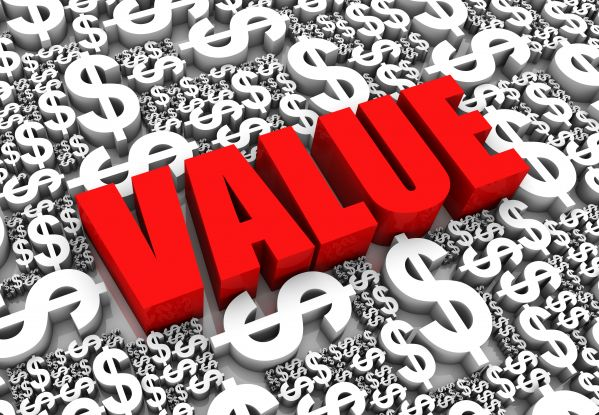# Converting Odds To Probability

I'm a maths geek. I really like probability. So when I make a betalways like to compare the probability of the result I am bettingon to the odds from the bookies. It is not for everyone but itgives a good

I'm a maths geek. I really like probability.So when I make a bet always like to compare the probability of the result I am betting on to the odds from the bookies.

It is not for everyone but it gives a good sense-check to whether you think the odds are reasonable and therefore offer a value betting opportunity.

The olbg betting school covers this in the brilliant how to become an expert in value betting.

### Example

Let's say you think Chelsea are going win the league and you think the chance of Chelsea retaining the title is at least 50%.

So what odds are you looking for to make it a worthwhile bet? The answer is evens (1/1) and it is pretty obvious, but if you are not sure of how to calculate that then read on.

Anything worse than evens (1/1) and you are not getting a value bet BASED ON YOUR OPINION THAT CHELSEA HAVE A 50% CHANCE!

### How To Convert Probability to Odds

To convert probability to odds take the probability as a fraction, in the example above, 50% = 1/2, and now put the number at the top on the bottom, the number at the bottom on top and minus one from the whole thing: (2/1)-1 = 1/1 or evens.

Another example might be that you think something has at least 66% probability of happening. In this case, the fraction is 2/3, so for a decent bet, the odds should be at least: (3/2)-1 = 1/2.

Sometimes you'll want to do it the other way round: You think Djokovic is going to beat Federer in the Wimbledon final. The Serb is best odds with bookmakers at 5/6 to win.

Is this good value?

You can check the probability odds table here, this will assist your calculations.

### How To Convert Odds To A Probability

To convert the odds to a probability, put the bottom number on top and divide by the sum of the top and bottom number.

So for Djokovic (5/6) the bookies are effectively saying they think he has (6+5) = 6/11 = 54.5% chance of winning.

If you think he has more chance of winning than that then this bet represents good value to you.

If you do your betting in decimals it is even easier. Just divide 1 by the decimal odds for the probability. And if you are going from probability to decimals, now you just divide 1 by the probability.

And that's all there is to it.

But if your mates ask you what you are doing when you are tapping numbers into the calculator on your phone in the pub, don't tell them you are calculating probabilities. They will probably call you a geek.Hi, username! Logout